## Is there always an IRR?

Jul 26

Written by: Charles Flock
7/26/2016 12:26 PMXIRR is one of our most popular functions. In this article we look at some of the reasons why XIRR returns a NULL and why that's OK.
XIRR calculates the internal rate of return (IRR) for a series of irregular cash flows, meaning that the cash flows can be for any amount and occur on any date. The value returned by XIRR will return a net present value (NPV) of 0 when those same cash flows are passed into the XNPV function using the XIRR value as the discount rate. Symbolically: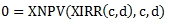Where

 XNPV: XNPV function XIRR: XIRR function c: vector of cash flow amounts d: vector of associated dates

Understanding the value returned by XIRR requires understanding the XNPV function. XNPV calculates the sum of the discounted cash flows using the supplied discount rate. Assuming that the cash flows are in ascending date order: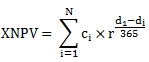Where

 N = number of cash flows ci = cash flow amount di = date of the cash flow r = 1 + discount rate

There are a couple of properties of the XNPV function that are worth pointing out. First, the discount rate must be greater than -1 otherwise we end up trying to raise a negative number to a fractional power, which is an illegal operation (Excel users should take note that Excel actually requires that the discount rate be greater than 0 rather than greater than -1 for no apparent reason).
Second, because XNPV is a summation of the discounted cash flows, all cash flows for the same date use the same fractional power and all the cash flows for the first date are always included in the summation at their full value, because the exponent is 0 making r0 = 1.
As we said in the first paragraph, XIRR seeks a value for the discount rate such that the XNPV is zero. This is exactly the same as saying that the sum of all the discounted cash flows where di > d1 is equal and opposite in value to the sum of the cash flows where di = d1. The discounted cash flow for each di has the same sign as the sum of the cash flows for the di as discounting will not change the sign. To calculate an XIRR, there must be at least one di where the sum of the cash flows is positive and one di where the sum of the cash flows is negative. Simply having positive and negative cash flow amounts is not enough, it's the summation by day that matters. This can be tremendously confusing for Excel users, as can be seen in the following example.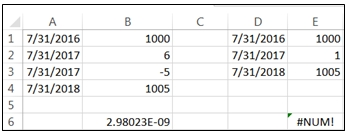Notice that in B6 Excel returns 2.98023E-09 (approximately zero), while in E6 the returned value is #NUM!, even though the cash flow amounts by day are exactly the same. Obviously, there is no calculable internal rate of return, despite the value in B6, as the sum of the cash flows for each date is positive.
This is the most common reason that the internal rate of return cannot be calculated: the sums of the cash flows for each date have the same sign.
Let's look at another situation. Let's say that you invest 100 on 2016-07-25 and on 2016-07-26 that 100 has turned into 700. While this might seem extreme, it's easy to imagine lots of scenarios (gambling, highly leveraged investments, options trading) where this kind of thing could happen. We could enter this in the following way into Google Sheets (or Excel).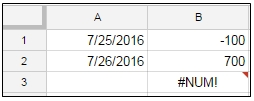In cell B3 we have entered our XIRR formula and it returns #NUM!. We have one positive and one negative cash flow. Why is NUM#! being returned?
The answer has to do with the limits of floating point math. Calculating the XIRR using extended precision mathematics (in other words, not IEEE 754), we calculate an XIRR of approximately 2.9e+308. The maximum value that can be represented in double precision floating point math is 1.7976931348623157E+308. Since the solution is greater than this value, it cannot be calculated within the constraints IEEE 754. The following chart shows the relationship between the XNPV values and the discount rates (which have been converted to their natural logs for ease of viewing) and you can see that we just cannot generate a value for discount rate big enough to get the XNPV to zero.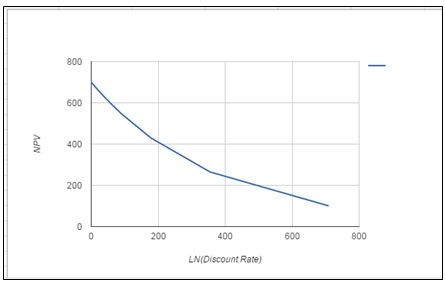Let’s look at another example.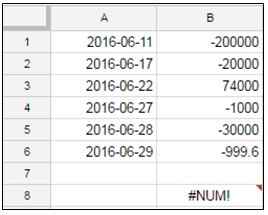In this example we have both positive and negative cash flows, yet no IRR can be calculated. Let’s look into why that is. Here's a graph of the NPV values.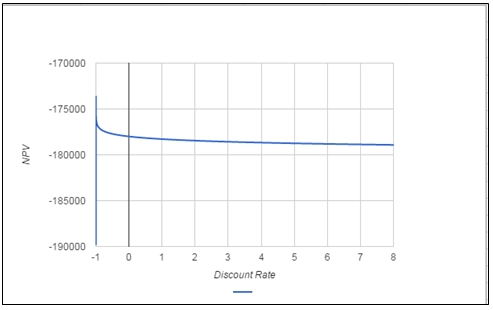Remember that we cannot have a discount rate less than or equal to -1. On the left side of the graph you can see a vertical line between -190,000 and -175,000. This represent all the NPV values calculated between -0.9999999999999999 and -0.99. You can then see that at -0.99 and greater that the NPV settles at around -177,000. There seems to be no value for the discount rate that brings the NPV close to zero, hence it is not possible to calculate an internal rate of return.
In XLeratorDB, when there is no solution for the XIRR, the function returns a NULL. In Google Sheets, #NUM! is returned. Excel sometimes returns #NUM! and sometimes returns 2.98023E-09 (so you need to be extremely careful when using the Excel function as it also returns 2.98023E-09 when, in fact, there is an easily found solution – see Comparing the Excel XIRR function to Google and XLeratorDB from 4/10/2015 for more).
Thus, an XIRR of NULL is a valid return value. It is not necessarily an indication of a problem of any kind and thus the treatment of NULL values should be incorporated into whatever reporting processes use the XIRR function.

You can try out the XLeratorDB XIRR function by downloading the free XLeratorDB 15-day trial. If you are not a SQL Server user, you can try out the XIRR function in your .NET programs by downloading the XLeratorDLL free 15-day trial.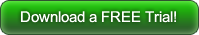exactly 2.8892465622275630164588776464796e+308. Since there are only 2 cash flows, it's relatively easy to see that the discount rate is the value that converts the 700 cash flow to 100 using this formula:
100 = POWER(1+r, -1/365)

Tags:
Categories:
Location: BlogsThe WestClinTech Blog

## Search Blogs

 KeywordsPhrase

## Blog Archives

Archive
 < September 2023 >
SunMonTueWedThuFriSat
272829303112
3456789
10111213141516
17181920212223
24252627282930
1234567
Monthly
Go

### SupportCopyright 2008-2023 Westclintech LLC         Privacy Policy        Terms of Service